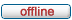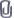All times are UTC

Forum rules

1. This forum can be used for discussion of both ALGLIB-related and general numerical analysis questions
2. This forum is English-only - postings in other languages will be removed.

 Print view Previous topic | Next topic
Author Message
 Post subject: minlmoptimize doesnt do anything! Help please!Posted: Thu Jun 11, 2020 4:56 pmJoined: Thu Jun 04, 2020 3:29 pm
Posts: 2
Hello,

I am trying to fit a curve of the form:
f(x)=c0+c1*tanh(c2*(x-c3))
to 300 points of 2d data e.g. "x" and "y" coordinates, in my case "x" is index and "y" is some given known numerical value.
I need this to be done fast and so after solving this with lsfit I tried to implement using minlm.
Since minlm minimizes a function of sum of squares form i.e.,
F=f1^2+f2^2+...fm^2
I defined my functions to be the errors of the data for a given guess of coefficients i.e.
fi=c0+c1*tanh(c2*(i-c3))-data(i)
and analytically computed the jacobian matrix (300X4).
Unfortunately, minlmresults seems to do nothing and I cant figure out why. Please help me, I have nowhere else to turn.
I post my code below and attach my data in a txt file. "esf" is the 300 points of data I mentions:

using System;
using System.IO;
using System.Text;
using System.Linq;

namespace minlm_d_vj_example
{
class Program
{
public static int Main(string[] args)
{
string[] esf_string_split = esf_string.Split('\n');
double[] esf = new double[esf_string_split.Length-1];

double[] ci = new double; //In this block I initialize the coefficients with a clever guess
ci = (esf + esf[^1]) / 2;
ci = (esf - esf[^1]) / 2;
int d = 0;
double dThreshold = ci;
for (; (d < esf.Length) && (esf[d] > dThreshold); d++) ;
ci = d;
int di = 2;
double f1 = esf[d - di];
double f2 = esf[d + di];
ci = -(f1 - f2) / (2 * di) / ci;

double[] s = new double[] { 1, 1, 1, 1};
double epsx = 0.0000000001;
int maxits = 0;

alglib.minlmstate state;
alglib.minlmcreatevj(esf.Length, ci, out state);
alglib.minlmsetcond(state, epsx, maxits);
alglib.minlmsetscale(state, s);
alglib.minlmoptimize(state, function1_fvec, function1_jac, null, null);
alglib.minlmreport rep;
alglib.minlmresults(state, out ci, out rep);
System.Console.WriteLine("{0}", alglib.ap.format(ci, 2));
alglib.optguardreport ogrep;
alglib.minlmoptguardresults(state, out ogrep);
return 0;

void function1_fvec(double[] c, double[] fi, object obj)
{
for (int i = 0; i < esf.Length; i++)
{
fi[i] = c + c * Math.Tanh(c * (i - c)) - esf[i];
}
}
void function1_jac(double[] c, double[] fi, double[,] jac, object obj)
{
for (int i = 0; i < esf.Length; i++)
{
fi[i] = c + c * Math.Tanh(c * (i - c)) - esf[i];
double k = i - c;
jac[i, 0] = 1; //Here I used some algebra to avoid unnecessary computations. OptGuard gives True but I can't find any problems with the jacobian.
jac[i, 1] = Math.Tanh(c * k);
jac[i, 2] = c * (1 - jac[i, 1] * jac[i, 1]) * k;
jac[i, 3] = jac[i, 2] * (-c / k);
}
}
}
}
}

 Attachments: File comment: This files contains the data to be fitted as a function of index.4_1_1_esf.txt [2.64 KiB] Downloaded 119 times
TopDisplay posts from previous: All posts1 day7 days2 weeks1 month3 months6 months1 year Sort by AuthorPost timeSubject AscendingDescending

 All times are UTC

Who is online

Users browsing this forum: No registered users and 2 guests

 You cannot post new topics in this forumYou cannot reply to topics in this forumYou cannot edit your posts in this forumYou cannot delete your posts in this forumYou cannot post attachments in this forum

Search for: